Cart# kW to Amps Calculator

By Benjamin Strusnik February 13, 2023

When you’re dealing with solar power and electrical equations, you’re going to run into terms like kW (kilowatts) and amps pretty frequently. It might not look like it at first, but these two values are interlinked and you can convert from one form to the other provided you have the right information.

Whether it's the output of your solar power system or the rating of your battery, knowing how to use a kW to amps calculator will help you understand the relationship between the units and components in your system.

Taking the time to understand energy terminologies is a good way to ensure that the decisions you make regarding solar or electrical power are carefully thought through.

## What Are Kilowatts?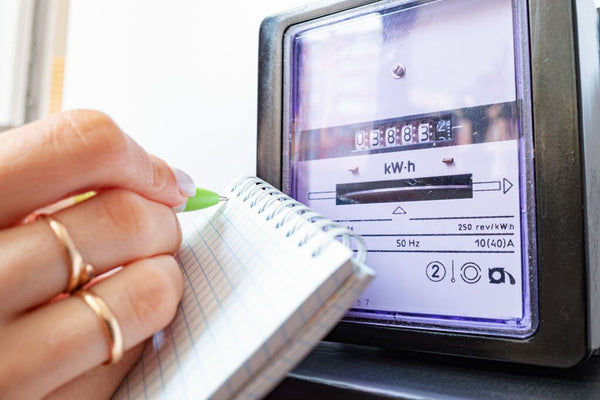A kilowatt is a measure of electrical power equivalent to 1,000 W.

When we speak about power, we’re referring to the rate at which appliances consume energy.

Watts are obtained by multiplying voltage and current according to Watt’s Law. When we multiply the resulting watts by 1,000, we get kilowatts.

We also use watts and kilowatts in solar equations to measure how much electricity the system produces.

If we have an array of portable solar panels for home use rated at 2 kW, this means that on a perfectly sunny day, the maximum output of this solar system is 2 kW, though it will regularly produce less than that.Provided we understand this concept, using a kW to kWh calculator is simple.

In this equation, all we do is multiply the kilowatts of a circuit by the time it’s operational to find the kWh.

## What Are Amps?

When electrons flow through a circuit, we get an electric current.

This current is measured in amps, short for amperes, which is an indication of the number of electrons flowing per second.

### Amps in a Circuit

Let’s say we have a simple circuit consisting of copper wire, a battery, and a lightbulb.

Electrons flow from the negative end of the battery through the copper wire. This then goes through the lightbulb to the positive side of the battery, completing the circuit.

When current flows in the circuit, all the electrons move simultaneously.

The battery’s negative terminal pushes the negative electrons away, which causes the current to flow.

If the circuit is broken, the current stops flowing and the electrons can’t jump the gap.

One of the things you need when you’re setting up your solar system is a fuse.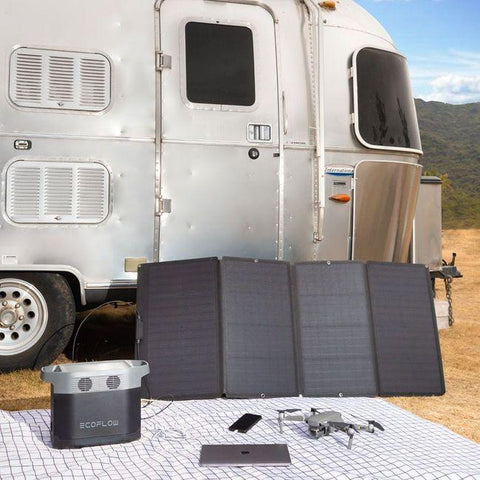If we have a 15 A fuse with 5 A flowing through it, the fuse can do its job and ensure that the current is protected.

The minute we start to draw 45 A from our 15 A fuse it melts and the circuit breaks.

Before we can look at how to convert kWh to kVA or figure out how to use a kW to amps calculator, we need to distinguish between conventional current and electron flow.

### Conventional Current Vs. Actual Electron Flow

Current flows from positive to negative. This is what electrical engineers call “conventional current”. However, the actual flow of electrons goes from negative to positive.

If we’re doing any calculations or conversations, we use conventional current. But the reality is current flows from negative to positive.

Now that we’re clued up on both of the variables in our calculator, we can look at how to do these calculations ourselves.

## How to Use a kW to Amps Calculator

Using a kW to amps calculator has a few more steps than a simple kWh per square foot calculator. For our calculation, you need to know two variables and there’s only one direct approach to calculating the answer.

One of the things that can make this topic so complicated is that there are three different formulas for different scenarios, and it’s not always clear when you’re supposed to use one over the other.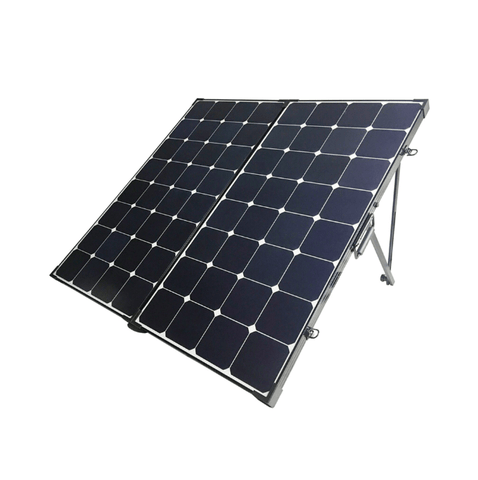The first is converting kW to amps for DC, then single-phase AC, and finally three-phase AC.

### Formula 1

The first formula is used in DC circuits and states that the current, or amperage, equals the kilowatts multiplied by 1,000, divided by the voltage:

I (DC) = (P (kW) x 1,000) / V

If we have a solar system rated at 5 kW with a 100 V DC motor powering it, what is the current flowing through the system?

Well, if we use our formula and substitute in the given values, we have 5 kW multiplied by 1,000, divided by 100, which gives us 50 A:

I = (5 x 1,000) / 100

I = 5,000 / 100

I = 50 A

This formula takes the given kW and converts it into the unknown amperage.

This also serves as a kWh to amp calculator as we can remove time as a variable from that calculator if we don’t need it in our final answer.

### Formula 2

The second formula we’re going to look at is used in single-phase AC circuits. Single-phase circuits rely on one neutral wire and one power wire.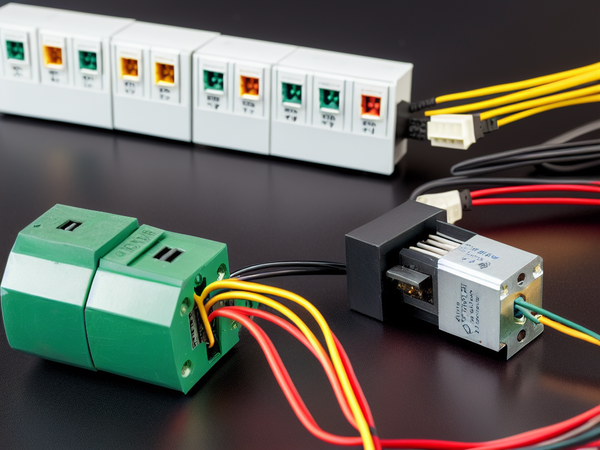For this formula states we multiply the power, in kilowatts, by 1000 and divide that by the product of the voltage and the power factor to get the current.

Let’s say we want to find the current flowing through a 5 kW solar system connected to a 230 V single-phase AC motor. The motor operates at a power factor of 0.8.

If we plug in the values correctly, the equation looks like this:

I = (5 x 1,000) / (230 x 0.8)

I = (5,000) / (184)

I = 27.17 A

This formula is like the DC circuit one but we’re accounting for the power factor which is how effective the appliance is at converting the voltage of a current into real work.

If your system is rated in watts, don’t panic. The kWh to watts conversion is easy since all you need to do is multiply or divide by 1,000.

### Formula 3

Finally, we have a kW to amps calculator in a three-phase AC circuit. Three-phase circuits have 3 power wires that carry the load.

In a three-phase AC circuit, current is equal to the kilowatts of the system multiplied by 1,000, divided by the product of the voltage, power factor, and the constant 1.73, or the approximate square root of 3 to be exact.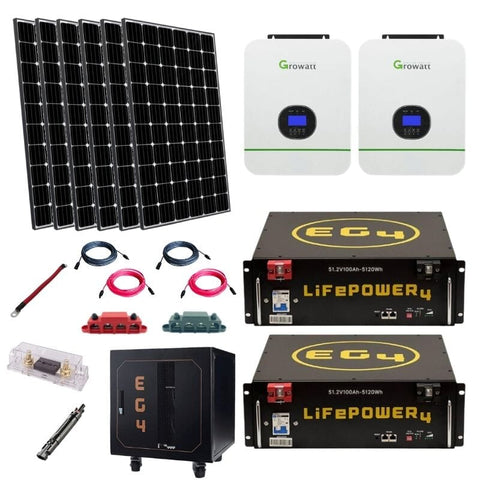To get a better understanding of how this calculator works, let’s look at a more practical example.

We want to find the current flowing through a 5 kW solar system hooked up to a 230 V three-phase AC motor that operates at a power factor of 0.9.

When we substitute the values into the equation, we’re left with 5 kW multiplied by 1,000 as the numerator, and the product of 230 V, 0.9, and 1.73 as the denominator.

Our equation looks like this:

I = (5 x 1,000) / (230 x 0.9 x 1.73)

I = (5,000) / (358.11)

I = 13.96 A

## Conclusion

While this is definitely a more complicated calculation than what you’ll find in a basic energy use calculator, the fundamentals behind the math are the same. Once you’ve worked out exactly how all these variables are related, you can figure out pretty much any component of your circuit provided you have some information to start off with.

The world of solar is filled with calculators and conversions. The more you know, the more confident you’ll feel setting up and maintaining your solar panel setup and all the relevant equipment.

Did You Find Our Blog Helpful? Then Consider Checking:Someone purchased a

#### EcoFlow Delta 1,800W / 1,300wH [Quad Kit] +...

26 minutes agoSomeone purchased a

#### Bluetti AC200P 2,000W / 2,000wH [Hex Kit] +...

26 minutes agoSomeone purchased a

#### Complete Off-Grid Solar Kit - MPP LV2424 2,400W...

26 minutes agoSomeone purchased a

#### Complete Off-Grid Solar Kit - 4,000W 120/240V Output...

26 minutes agoSomeone purchased a

#### Complete Off-Grid Solar Kit - 6,000W 120/240V Output...

26 minutes agoSomeone purchased a

#### EcoFlow DELTA PRO 3,600wH / 3,600W [Hex Kit]...

26 minutes ago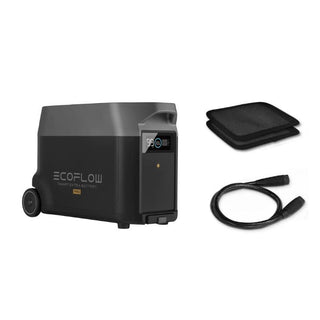Someone purchased a

#### EcoFlow DELTA PRO [Extra Battery] | 3,600wH Capacity...

26 minutes agoSomeone purchased a

#### EcoFlow DELTA [PRO] 3,600wH / 3,600W [NOMAD Kit]...

26 minutes agoSomeone purchased a

#### EcoFlow DELTA PRO 3,600wH / 3,600W Solar Generator...

26 minutes agoSomeone purchased a

#### Complete DIY Solar Panel Kit - 3,000W Inverter...

26 minutes agoSomeone purchased a

#### Complete Solar Kit - 7,200W 120/240V Output /...

26 minutes agoSomeone purchased a

#### Complete Off-Grid Solar Kit - 6,000W 120/240V Output...

26 minutes agoSomeone purchased a

#### Complete Off-Grid Solar Kit - 6,000W 120/240 Output...

26 minutes agoSomeone purchased a

#### EcoFlow DELTA PRO 3,600wH / 3,600W [Quad Kit]...

26 minutes agoSomeone purchased a

#### Complete DIY Solar Panel Kit - 2,000W Pure...

26 minutes agoSomeone purchased a

#### Complete Hybrid Solar Kit - Sol-Ark 12K 120/240V...

26 minutes agoSomeone purchased a

#### Complete Off Grid Solar Kit - 3,000W /...

26 minutes agoSomeone purchased a

#### Complete Off-Grid Solar Kit - 13,000W 120/240V Output...

26 minutes agoSomeone purchased a

26 minutes ago